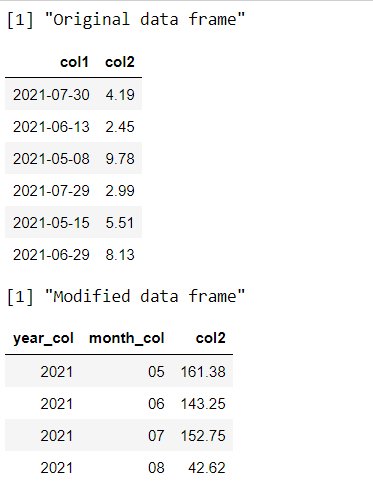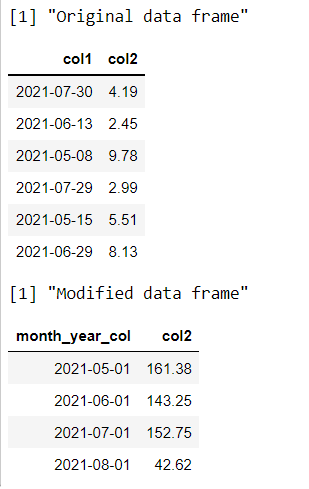Aggregate Daily Data to Month and Year Intervals in R DataFrame

• Last Updated : 23 Sep, 2021

In this article, we are going to see how to aggregate daily data over a period of months and year intervals in dataframe in R Programming Language.

Method 1 : Using aggregate() method

Base R contains a large number of methods to perform operations on the dataframe. The seq() method in R is used to generate regular sequences beginning from a pre-defined value.

Syntax: seq(from , to , by , length.out)

Arguments :

• from – The value from where to begin the sequence. The as.Date() method is used here, in order to generate a sequence of dates until the length of the sequence is met.
• to – The value where to end the sequence.
• by – The parameter to increment the sequence. “day” is used as a parameter here, in order to generate successive dates in order.
• length.out – The total length of the sequence.

The dataframe is then formed using a sample from this date sequence generated as column 1. The value is generated using the rnorm() method to produce random floating-point numbers.

The strftime() method is then used to convert a time object to a character string back. The format can be specified to extract different components of the date object.

Syntax: strftime (date, format)

Arguments :

• date – The object to be converted
• format – We use %m to extract the month and %Y to extract the year in YYYY format.

In order to aggregate the data, the aggregate method is used, which is used to compute summary statistics of each of the groups.

Syntax: aggregate ( formula , data , FUN)

Arguments :

• formula – a formula, such as y ~ x
• data – The dataframe over which to apply the function
• FUN – The function to be applied to the dataframe.  Here, the function applied is sum in order to perform the aggregation or summation over the values belonging to same group.

Code:

R

 set.seed(99923)     # creating dataframe # specifying number of rows len <- 100    # creating sequences of dates var_seq <- seq(as.Date("2021/05/01"),                by = "day",                length.out = len)    # creating columns for dataframe data_frame <- data.frame(col1 = sample( var_seq,                                  100, replace = TRUE),                    col2 = round(rnorm(10, 5, 2), 2)) print("Original dataframe") head(data_frame)    # creating new year column for dataframe data_frame\$year_col <- strftime(data_frame\$col1, "%Y")       # creating new month column for dataframe data_frame\$month_col <- strftime(data_frame\$col1, "%m")    # aggregating the daily data data_frame_mod <- aggregate(col2 ~ year_col + month_col,                                data_frame,                         FUN = sum) print("Modified dataframe") head(data_frame_mod)

Output:Method 2: Using lubridate and dplyr package

Lubridate package in R is used to provide easier working mechanisms with the date and time objects. It can be loaded and installed in the working space using the following command :

install.packages("lubridate")

The floor_date() method in R uses a date-time object, may be a single entity or vector of date-time objects, and then further rounds it off to the nearest integer value in the specified unit of time.

floor_date(x , unit = months)

The dplyr package is used to perform data manipulations and statistics. It can be loaded and installed in the working space using the following command :

install.packages("dplyr")

The dataframe is modified by using the piping operator over a sequence of operations and methods. The group_by() method is used to group the data based on the values contained in the specified columns.

group_by(col1,..)

Then a summary statistic is performed using the summarize() method which performs a summation over the values contained in the third column. The result is then manipulated into a dataframe using the as.data.frame() method.

Code:

R

 library("dplyr") library("lubridate") set.seed(99923)     # creating dataframe # specifying number of rows len <- 100    # creating sequences of dates var_seq <- seq(as.Date("2021/05/01"),                by = "day",                length.out = len)    # creating columns for dataframe data_frame <- data.frame(col1 = sample( var_seq,                                  100, replace = TRUE),                    col2 = round(rnorm(10, 5, 2), 2)) print("Original dataframe") head(data_frame)    # creating new month column for dataframe data_frame\$month_year_col <- floor_date(data_frame\$col1,                                     "month") # aggregating the daily data data_frame_mod <- data_frame %>%                           group_by(month_year_col) %>%    dplyr::summarize(col2 = sum(col2)) %>%    as.data.frame()    print("Modified dataframe") head(data_frame_mod)

Output:My Personal Notes arrow_drop_up
Recommended Articles
Page :